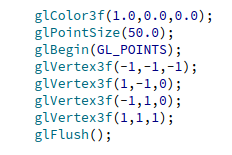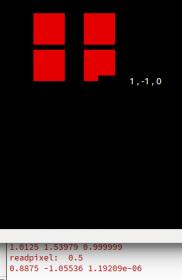# Select points with mouse in 2D space?

I use OpenGL to draw objects, lines, points, etc. in 3D space. Ofcourse, this gets displayed on a 2D screen. Now I want to be able to select the nearest 3D point by clicking the mouse near a 3D point. Any idea how to do this? I programming in QtCreator and i use QPoint to get (x,y) the coordinates of the mouse in th windows. How to get z position?I tryed used glReadPixels() for obtain z cooridnate. but, always returns 0. This is my function:

``````GLfloat depth = 0.0f;
glReadPixels(x, glutGet( GLUT_WINDOW_HEIGHT ) - y, 1, 1, GL_DEPTH_COMPONENT, GL_FLOAT, &depth);
``````

Why are you calling GLUT functions in a Qt application? That won’t work if you didn’t use GLUT to create the window.

Yes, I just realized that I was using GLUT. For the creation of the window I am using openGL in Qt with c ++. Sorry for my english, it’s not goodThis is my method of init:

``````void
MeshViewer::initializeGL()
{
GLfloat LightAmbient= { 0.7f, 0.7f, 0.7f, 1.0f }; // Ambient Light Values ( NEW )
GLfloat LightDiffuse= { 1.0f, 1.0f, 1.0f, 1.0f };	// Diffuse Light Values ( NEW )
GLfloat LightPosition= { 0.0f, 0.0f, 2.0f, 1.0f };	// Light Position ( NEW )

//setupViewport(width(), height());
glViewport(0, 0, (GLint)width(), (GLint)height());

qglClearColor(Qt::black);
glClearDepth(1.0);
glEnable(GL_DEPTH_TEST);
glDepthFunc(GL_LEQUAL);
glHint(GL_PERSPECTIVE_CORRECTION_HINT, GL_NICEST);
glEnable(GL_CULL_FACE);
glEnable(GL_LIGHTING);
glEnable(GL_LIGHT0);
glEnable(GL_MULTISAMPLE);

glHint(GL_PERSPECTIVE_CORRECTION_HINT, GL_NICEST);

glLightfv(GL_LIGHT0, GL_AMBIENT, LightAmbient);
glLightfv(GL_LIGHT0, GL_DIFFUSE, LightDiffuse);
glLightfv(GL_LIGHT0, GL_POSITION,LightPosition);
glEnable(GL_TEXTURE_2D);

//to enable my_own_blend effect
glEnable(GL_COLOR_MATERIAL);

glBlendFunc(GL_SRC_ALPHA, GL_ONE_MINUS_SRC_ALPHA);
glEnable(GL_BLEND);
}
}
``````

In which case, you need:

``````
glReadPixels(x, height() - 1 - y, 1, 1, GL_DEPTH_COMPONENT, GL_FLOAT, &depth);

``````

An OpenGL context needs to be bound when calling any OpenGL functions. This is done automatically for the paintGL() method of a QOpenGLWidget, but if you’re making OpenGL calls from other functions, you may need to call makeCurrent() explicitly.

[QUOTE=GClements;1292351]In which case, you need:

``````
glReadPixels(x, height() - 1 - y, 1, 1, GL_DEPTH_COMPONENT, GL_FLOAT, &depth);

``````

An OpenGL context needs to be bound when calling any OpenGL functions. This is done automatically for the paintGL() method of a QOpenGLWidget, but if you’re making OpenGL calls from other functions, you may need to call makeCurrent() explicitly.[/QUOTE]

Okay thanks, works perfectly. Depth return other values, but i have a question. I am use x and y as the mouse position in the window and glReadPixels it is activated when i click. How can i verify that the value returned by the variable depth corresponds to position Z?

Fill the screen with a quad (or triangle pair), with vertices at (-1,-1,-1), (1,-1,0), (-1,1,0), (1,1,1) in NDC (i.e. with both model-view and projection matrices set to an identity matrix). Then it’s trivial to compute what the depth should be for a given (x,y) position: depth=((x+0.5)/width+(y+0.5)/height)/2.

Thanks! I did what you told me and I get the Z value with the method glReadPixels(); but with ur equation the values ​​differ a bit.
Example, with glReadPixels when I click in the windows to get Z coordinate i get for example z=0.495, and with ur equation depth=((x+0.5)/width+(y+0.5)/height) i get z=0.51 or or something similar. It is right? Can I assume that small margin of error as correct?

Now, in my program i have this in the method

``````
/* Detremining the window z from glReadPixels() */
GLfloat z = 0.0f;
glReadPixels(lastPos.x(), height() - 1 - lastPos.y(),1, 1,
GL_DEPTH_COMPONENT, GL_FLOAT, &z);

/*Get x, y, z coordinates of points in the window*/
GLdouble modelView;
glGetDoublev(GL_MODELVIEW_MATRIX, modelView);
GLdouble projection;
glGetDoublev(GL_PROJECTION_MATRIX, projection);
GLint viewport;
glGetIntegerv(GL_VIEWPORT, viewport);
GLdouble wx,wy,wz;

/*lastpos it's a QPoint variable. lastpost.x() get X position of the mouse in the window. and y the same.*/
gluUnProject(lastPos.x(),viewport - 1 - lastPos.y(),z,modelView,projection,viewport,
&wx,&wy,&wz);

qDebug() << wx << wy << wz

``````

[QUOTE=pelaoqlo;1292376]Thanks! I did what you told me and I get the Z value with the method glReadPixels(); but with ur equation the values ​​differ a bit.
Example, with glReadPixels when I click in the windows to get Z coordinate i get for example z=0.495, and with ur equation depth=((x+0.5)/width+(y+0.5)/height) i get z=0.51 or or something similar. It is right? Can I assume that small margin of error as correct?
[/QUOTE]
Errors shouldn’t be more than a small multiple of 1/2n, where n is the number of bits in the depth buffer (typically either 16 or 24).

First, you should be adding 0.5 to the x and y coordinates, as the depth value is calculated for the centre of the pixel, not its lower-left corner. Window coordinates which are integers correspond to pixel corners; window coordinates with a fractional part of 0.5 correspond to pixel centres. I.e.:

``````
gluUnProject(lastPos.x() + 0.5, viewport - 0.5 - lastPos.y(), ...);

``````

Second, if you’re using a perspective projection, the accuracy of depth values can vary wildly depending upon the distance from the near plane. Roughly half of the available depth values are used for -Z values between the near distance and twice the near distance. More generally, 1/N of the available depth values are used for -Z values beyond N times the near plane. If the ratio of far distance to near distance is large, most of the available depth values are used for a relatively small portion of the scene.

To check the accuracy, un-project the window-space x,y coordinates with both z=0 and z=1 to get two points on a line in object space. Find the intersection of that line with the plane x+y-2*z=0, project the intersection point back to window space, and compare the window-space Z to the value in the depth buffer.

I am using orthogonal projection for my program, and this is my resize method:

`````` void
MeshViewer::resizeGL(int width, int height)
{
if ( height == 0 )
height = 1;
glViewport(0,0,(GLsizei)width,(GLsizei)height);
glMatrixMode(GL_PROJECTION);
glOrtho(xmin, xmax, ymin, ymax, zmin, zmax);
glMatrixMode(GL_MODELVIEW);
}
``````

x, y, z, they are the maximum and minimum values of the coordinates that I am visualizing in my program, for example if I have a list of coordinates: (-1,2,-1) , (-2,5,0), (2,1,1).

xmin = -2, xmax = 2
ymin = 1, ymax = 5
zmin = -1, zmax = 1Am I performing the procedure correctly to obtain the value of Z?
So, I think I’m correctly obtaining the position of the coordinates in the window (?)

Hi!

You could avoid using glReadPixels() altogether, why not just cast a ray into the scene and check which triangle it hits? In the simplest case, you intersect all objects in the scene with the ray and take the one with the smallest distance. As your scene gets larger, this linear search will get inefficient, and you will have to construct a bounding volume hierarchy for your objects (e.g. a binary AABB tree). Once the hierarchy is created, you can perform kind of a binary search to find the closest intersection whenever you cast a ray. I’m aware that this solution requires some effort to implement, but it’s robust and scalable.

Here is some code to construct a ray in world space:

``````
auto ndc = mouse_pos_uv * 2.f - 1.f; // mouse_pos_uv are mouse coordinates between 0 and 1
auto near_h = inverse(view_projection_matrix) * Vec4f(ndc, -1.f, 1.f);
auto far_h = inverse(view_projection_matrix) * Vec4f(ndc, 1.f, 1.f);
auto near = near_h.xyz() / near_h.w();
auto far = far_h.xyz() / far_h.w();
auto ray_origin = near;
auto ray_dir = normalize(far - near);

``````

And here is how to intersect a ray with a box:

``````
static inline auto rayIntersectsBoundingVolume(const AABB& aabb, const Ray& ray)
{
auto t1 = (aabb.getMin() - ray.getOrigin()) * ray.getInvDir();
auto t2 = (aabb.getMax() - ray.getOrigin()) * ray.getInvDir();
float t_min = maxReduce(minimum(t1, t2));
float t_max = minReduce(maximum(t1, t2));
return std::tuple<bool, float>(t_min <= t_max && t_max >= 0.f, t_min);
}

``````